# Slope-Intercept Form of a Line

Slope-Intercept Form of a Line

Lines parallel to one of the two axes are special cases; now, we want to find the equation of a line with an arbitrary inclination. Visualize any such line in your mind. To completely specify such a line, you would need two quantities: the inclination of the line (or its slope or the angle it makes with say, the x-axis) and the placement of the line (i.e. where the line passes through with reference to the axes; we can specify the placement of the line by specifying the point on the y-axis through which the line passes, or in other words, by specifying the y-intercept).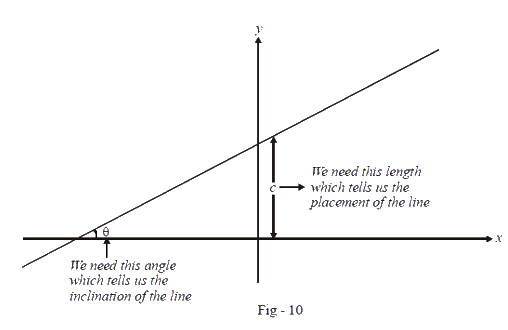It should be obvious to you that any line can be determined uniquely using these two parameters.

We now find out the equation of this straight line, assuming that we know $$\theta$$ and c.

What do mean by the phrase “equation of a straight line”? The equation of a straight line will be that relation which:

• the coordinates of any point on the line must satisfy

• the coordinates of any point not on the line will not satisfy

The determination of this equation is straightforward. Consider the following figure, in which a straight line is inclined at an angle of $$\theta$$ to the horizontal, and cuts the y-axis at $$A\left( {0,c} \right)$$ . $$P\left( {x,y} \right)$$ is an arbitrary point on this line. We need to find a relation which the coordinates of P satisfy. We have drawn AB parallel to the x-axis. By virtue of the fact that the inclination of a line is constant, we note that $$\angle PAB$$ is also theta: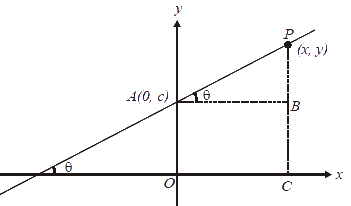In $$\Delta PAB$$ , we have:

$\tan \theta = \frac{{PB}}{{AB}}$

We have seen in trigonometry that the tan of a line’s angle of inclination is a measure of its steepness. The larger the tan, the steeper is the line. Thus, we term $$\tan \theta$$ the slope of the line, and denote it by m.

Next, we note that $$PB = y - c$$ and $$AB = x$$ . Thus,

\begin{align}&\tan \theta = m = \frac{{y - c}}{x}\\&\Rightarrow \,\,\,y - c = mx\,\,\, \Rightarrow \,\,\,y = mx + c\end{align}

This is the general equation of a straight line involving its slope and its y-intercept. This form of the equation of the line is therefore termed the slope-intercept form.

We note the following:

1.  A line may have negative slope – in case the angle it makes with the positive x-direction is an obtuse angle, as shown in the figure below: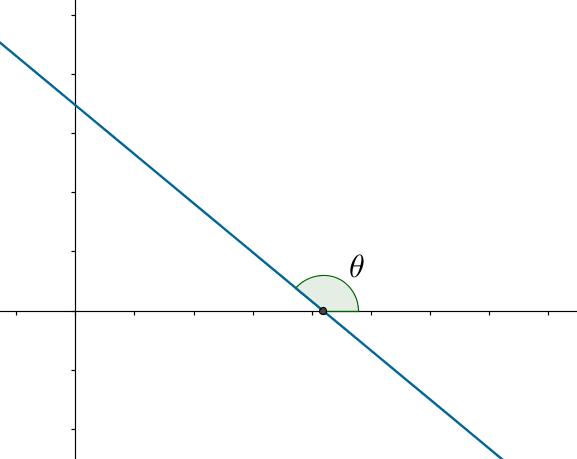The value of $$\tan \theta$$ in this case will be negative, so m will be negative.

2. The following figure shows a line passing through the origin: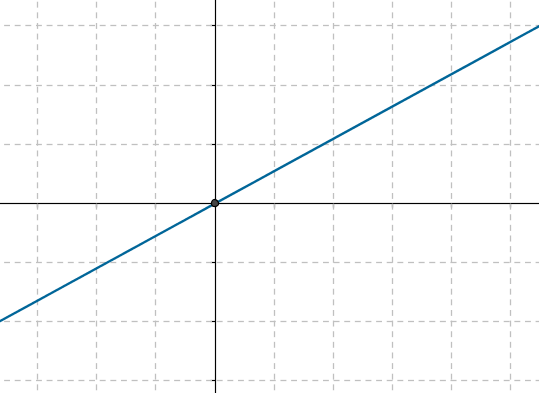For any such line, the y-intercept will be $$c = 0$$, so its equation will be of the form $$y = mx$$ .

Example 1: A line is inclined at an angle of 600 to the horizontal, and passes through the point $$\left( {0, - 1} \right)$$ . Find the equation of the line.

Solution: The following figure shows the specified line: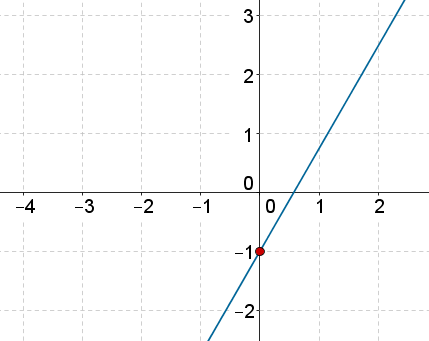We have

$m = \tan {60^0} = \sqrt 3$

Thus, the equation of the line is

$\begin{array}{l}y = mx + c\\ \Rightarrow \,\,\,y = \left( {\sqrt 3 } \right)x + \left( { - 1} \right)\\ \Rightarrow \,\,\,y = \sqrt 3 x - 1\end{array}$

Example 2: The equation of a line is $$3x + 4y + 5 = 0$$ . Determine the slope and y-intercept of the line.

Solution: We re-arrange the equation of the line to write it in the standard form $$y = mx + c$$. We have:

$\begin{array}{l}4y = - 3x - 5\\ \Rightarrow \,\,\,y = \left( { - \frac{3}{4}} \right)x + \left( { - \frac{5}{4}} \right)\end{array}$

Thus,

$m = - \frac{3}{4},\,\,\,c = - \frac{5}{4}$

Coordinate Geometry
Grade 9 | Questions Set 1
Coordinate Geometry